Test: Alligation or Mixture- 1

# Test: Alligation or Mixture- 1

Test Description

## 10 Questions MCQ Test CSAT Preparation for UPSC CSE | Test: Alligation or Mixture- 1

Test: Alligation or Mixture- 1 for Banking Exams 2023 is part of CSAT Preparation for UPSC CSE preparation. The Test: Alligation or Mixture- 1 questions and answers have been prepared according to the Banking Exams exam syllabus.The Test: Alligation or Mixture- 1 MCQs are made for Banking Exams 2023 Exam. Find important definitions, questions, notes, meanings, examples, exercises, MCQs and online tests for Test: Alligation or Mixture- 1 below.
Solutions of Test: Alligation or Mixture- 1 questions in English are available as part of our CSAT Preparation for UPSC CSE for Banking Exams & Test: Alligation or Mixture- 1 solutions in Hindi for CSAT Preparation for UPSC CSE course. Download more important topics, notes, lectures and mock test series for Banking Exams Exam by signing up for free. Attempt Test: Alligation or Mixture- 1 | 10 questions in 20 minutes | Mock test for Banking Exams preparation | Free important questions MCQ to study CSAT Preparation for UPSC CSE for Banking Exams Exam | Download free PDF with solutions
 1 Crore+ students have signed up on EduRev. Have you?
Test: Alligation or Mixture- 1 - Question 1

### 8 litres are drawn from a cask full of wine and is then filled with water. This operation is performed three more times. The ratio of the quantity of wine now left in cask to that of the water is 16:81. How much wine did the cask originally hold?

Detailed Solution for Test: Alligation or Mixture- 1 - Question 1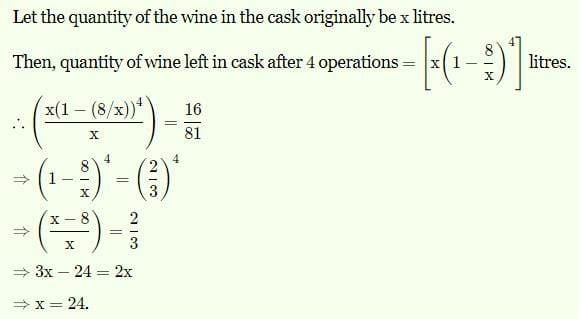Test: Alligation or Mixture- 1 - Question 2

### A container contains 40 litres of milk. From this container 4 litres of milk was taken out and replaced by water. This process was repeated further two times. How much milk is now contained by the container?

Detailed Solution for Test: Alligation or Mixture- 1 - Question 2

Assume that a container contains x of liquid from which y units are taken out and replaced by water. After n operations, the quantity of pure liquid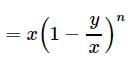Hence milk now contained by the container = 40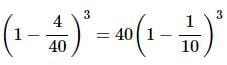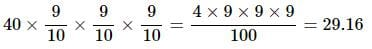Test: Alligation or Mixture- 1 - Question 3

### How many kilograms of sugar costing Rs. 9 per kg must be mixed with 27 kg of sugar costing Rs. 7 per Kg so that there may be a gain of 10 % by selling the mixture at Rs. 9.24 per Kg ?

Detailed Solution for Test: Alligation or Mixture- 1 - Question 3

► S.P. of 1 kg of mixture = Rs. 9.24, Gain 10%.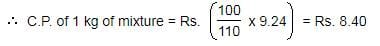By the rule of allilation, we have: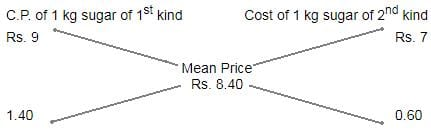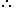Ratio of quantities of 1st and 2nd kind = 14 : 6 = 7 : 3.

► Let x kg of sugar of 1st be mixed with 27 kg of 2nd kind.

Then, 7 : 3 = x : 27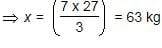Test: Alligation or Mixture- 1 - Question 4

A milk vendor has 2 cans of milk. The first contains 25% water and the rest milk. The second contains 50% water. How much milk should he mix from each of the containers so as to get 12 litres of milk such that the ratio of water to milk is 3 : 5?

Detailed Solution for Test: Alligation or Mixture- 1 - Question 4

► Let x and (12-x) litres of milk be mixed from the first and second container respectively

► Amount of milk in x litres of the the first container = .75x

► Amount of water in x litres of the the first container = .25x

► Amount of milk in (12-x) litres of the the second container = .5(12-x)

► Amount of water in (12-x) litres of the the second container = .5(12-x)

► Ratio of water to milk = [.25x + .5(12-x)] : [.75x + .5(12-x)] = 3 : 5

⇒ (.25x+6-5x)/(.75x+6-.5x) =3/5

⇒(6−.25x)/(.25x+6) =3/5

⇒30−1.25x=.75x+18

⇒2x=12

⇒x=6

► Since x = 6, 12-x = 12-6 = 6

Hence 6 and 6 litres of milk should mixed from the first and second container respectively

Test: Alligation or Mixture- 1 - Question 5

Two vessels A and B contain spirit and water in the ratio 5 : 2 and 7 : 6 respectively. Find the ratio in which these mixture be mixed to obtain a new mixture in vessel C containing spirit and water in the ration 8 : 5 ?

Detailed Solution for Test: Alligation or Mixture- 1 - Question 5

► Spirit in 1 litre mix of A = 5/7 litre.
► Spirit in 1 litre mix of B = 7/13 litre.
► Spirit in 1 litre mix of C = 8/13 litre.
By rule of alligation we have required ratio X:Y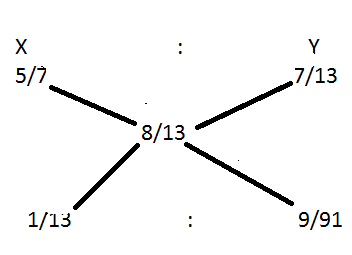Therefore required ratio = 1/13 : 9/91
= 7:9

Test: Alligation or Mixture- 1 - Question 6

Tea worth Rs. 126 per kg and Rs. 135 per kg are mixed with a third variety of tea in the ratio 1 : 1 : 2. If the mixture is worth Rs. 153 per kg, what is the price of the third variety per kg ?

Detailed Solution for Test: Alligation or Mixture- 1 - Question 6

► Since first and second varieties are mixed in equal proportions.So, their average price = Rs.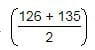= Rs. 130.50

► So, the mixture is formed by mixing two varieties, one at Rs. 130.50 per kg and the other at say, Rs. x per kg in the ratio 2 : 2, i.e., 1 : 1. We have to find x.

By the rule of alligation, we have: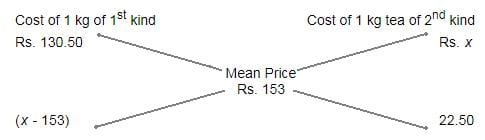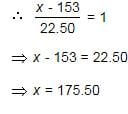Test: Alligation or Mixture- 1 - Question 7

A jar is full of whiskey contains 40% alcohol. A part of this whiskey is replaced by another containing 19% alcohols and now the percentage of alcohol was found to be 26%. The quantity of whiskey replaced is:

Detailed Solution for Test: Alligation or Mixture- 1 - Question 7

By the rule of alligation we have
► So ratio of 1st and 2nd quantities = 7:14
= 1:2
∴ Required quantity replaced = 2/3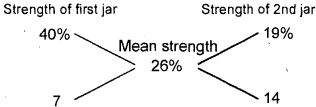Test: Alligation or Mixture- 1 - Question 8

The cost of Type 1 material is Rs. 15 per kg and Type 2 material is Rs.20 per kg. If both Type 1 and Type 2 are mixed in the ratio of 2 : 3, then what is the price per kg of the mixed variety of material?

Detailed Solution for Test: Alligation or Mixture- 1 - Question 8

► Cost Price(CP) of Type 1 material is Rs. 15 per kg
► Cost Price(CP) of Type 2 material is Rs. 20 per kg
Type 1 and Type 2 are mixed in the ratio of 2 : 3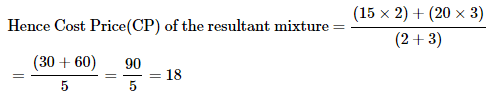Therefore, rice per kg of the mixed variety of material = Rs.18

Test: Alligation or Mixture- 1 - Question 9

Find the ratio in which rice at Rs. 7.20 a kg be mixed with rice at Rs. 5.70 a kg to produce a mixture worth Rs. 6.30 a kg.

Detailed Solution for Test: Alligation or Mixture- 1 - Question 9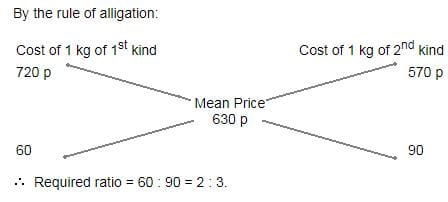Test: Alligation or Mixture- 1 - Question 10

In what ratio should rice at Rs.9.30 per Kg be mixed with rice at Rs. 10.80 per Kg so that the mixture be worth Rs.10 per Kg?

Detailed Solution for Test: Alligation or Mixture- 1 - Question 10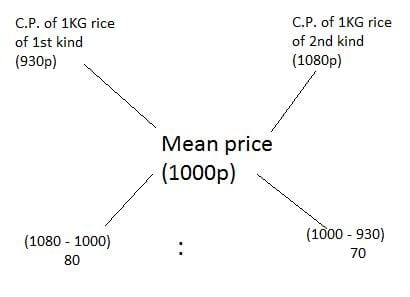Thus, required ratio = 80 : 70 = 8 : 7

## CSAT Preparation for UPSC CSE

72 videos|64 docs|92 tests
 Use Code STAYHOME200 and get INR 200 additional OFF Use Coupon Code
Information about Test: Alligation or Mixture- 1 Page
In this test you can find the Exam questions for Test: Alligation or Mixture- 1 solved & explained in the simplest way possible. Besides giving Questions and answers for Test: Alligation or Mixture- 1, EduRev gives you an ample number of Online tests for practice

## CSAT Preparation for UPSC CSE

72 videos|64 docs|92 tests